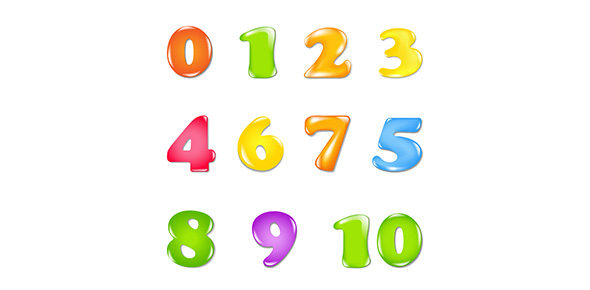# How Much Do You Know About Numbers?

Approved & Edited by ProProfs Editorial Team
At ProProfs Quizzes, our dedicated in-house team of experts takes pride in their work. With a sharp eye for detail, they meticulously review each quiz. This ensures that every quiz, taken by over 100 million users, meets our standards of accuracy, clarity, and engagement.
| Written by Samb
S
Samb
Community Contributor
Total Contribution - 4 | Total attempts - 46,134
Questions: 7 | Attempts: 121SettingsThink you are a numbers whiz? Prove it and take our brief quiz on numbers and number theory.

• 1.

### Which of the following numbers is a multiple of 3?

• A.

173191

• B.

222222

• C.

914333

• D.

333332

• E.

None of the above

B. 222222
Explanation
The number 222222 is a multiple of 3 because the sum of its digits (2+2+2+2+2+2) is equal to 12, which is divisible by 3.

Rate this question:

• 2.

### Which of the following numbers, when squared, is equal to the opposite of one?

• A.

-1

• B.

I

• C.

-i

• D.

Pi

• E.

-pi

B. I
Explanation
The number "i" is equal to the square root of -1. When "i" is squared, it becomes -1, which is the opposite of one. Therefore, "i" is the correct answer.

Rate this question:

• 3.

### Which of the following sets is larger: the set of real numbers or the set of complex numbers?

• A.

Real numbers

• B.

Complex numbers

• C.

Both are equally large

• D.

Both contain an infinite number of elements so it is impossible to determine largeness

• E.

They are the same set

B. Complex numbers
Explanation
The set of complex numbers is larger than the set of real numbers. This is because the set of complex numbers includes all real numbers as a subset, but also includes imaginary numbers. Imaginary numbers cannot be expressed as real numbers and are denoted by the square root of negative numbers. Therefore, the set of complex numbers is larger as it contains both real and imaginary numbers.

Rate this question:

• 4.

### If you add 1/4 + 1/5 + 1/6 ... indefinitely, you reach:

• A.

1

• B.

1/2

• C.

Pi

• D.

E

• E.

Infinity

E. Infinity
Explanation
When adding fractions such as 1/4, 1/5, 1/6, and so on, the denominators get larger and larger. As the denominators increase, the value of each fraction decreases. However, since the number of fractions being added is infinite, the sum will also be infinite. This is because no matter how small each fraction becomes, there will always be an additional fraction to add. Therefore, the sum of 1/4 + 1/5 + 1/6 ... indefinitely is infinity.

Rate this question:

• 5.

### Which is true regarding prime numbers?

• A.

As numbers increase the density of prime increases

• B.

They are a finite set

• C.

They exist for imaginary numbers

• D.

They are exclusive to the natural numbers

• E.

None of the above

D. They are exclusive to the natural numbers
Explanation
Prime numbers are exclusive to the natural numbers because they are positive integers greater than 1 that have no positive divisors other than 1 and themselves. They cannot be fractions, decimals, negative numbers, or zero. Therefore, prime numbers are a subset of the natural numbers and do not include any other types of numbers such as imaginary numbers or fractions.

Rate this question:

• 6.

### Which of the following represents the sum of the numbers between 1 and 100?

• A.

2000

• B.

15000

• C.

4525

• D.

5050

• E.

None of the above

D. 5050
Explanation
The sum of the numbers between 1 and 100 can be calculated using the formula for the sum of an arithmetic series, which is (n/2)(first term + last term). In this case, the first term is 1 and the last term is 100, so the sum is (100/2)(1 + 100) = 50(101) = 5050.

Rate this question:

• 7.

### Which of the following is true regarding division by zero?

• A.

It results in the imaginary number

• B.

It is undefined

• C.

It is equal to infinity

• D.

It is equal to zero

• E.

None of the aboveBack to top